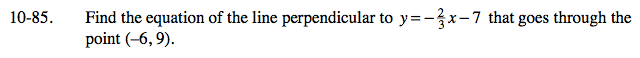### Home > CAAC > Chapter 10 > Lesson 10.2.4 > Problem10-85

10-85.Perpendicular lines have opposite, reciprocal slopes.

Substitute the given coordinates and the slope into the slope intercept form of a line to find b.

Rewrite the equation, substituting the values you just found for m and b.

$y=\frac{3}{2}x+18$